# 5 1 Equations n Solve equations using multiplication

• Slides: 46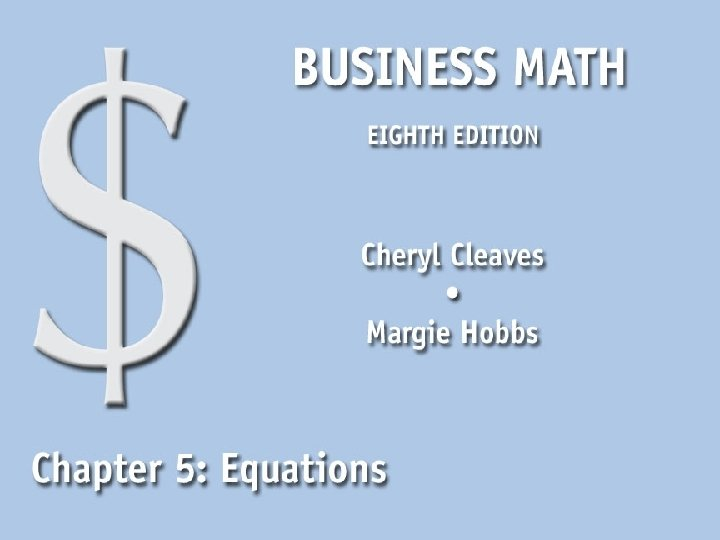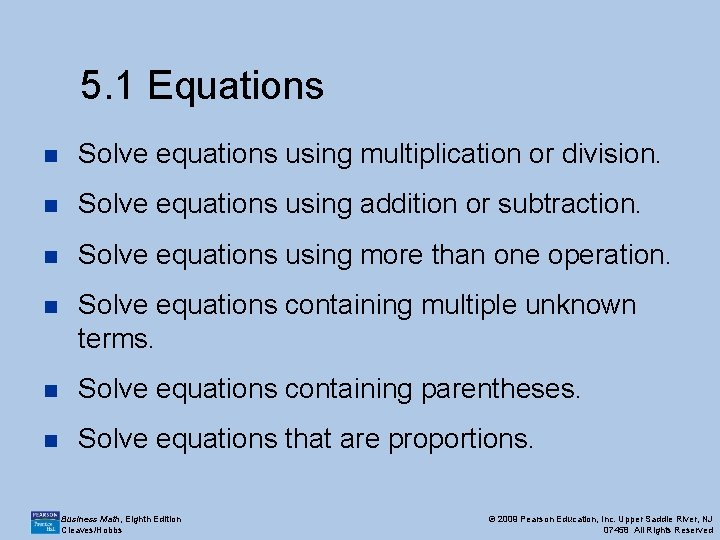5. 1 Equations n Solve equations using multiplication or division. n Solve equations using addition or subtraction. n Solve equations using more than one operation. n Solve equations containing multiple unknown terms. n Solve equations containing parentheses. n Solve equations that are proportions. Business Math, Eighth Edition Cleaves/Hobbs © 2009 Pearson Education, Inc. Upper Saddle River, NJ 07458 All Rights Reserved5. 1. 1 Solve Equations Using Multiplication or Division n An equation is a mathematical statement in which two quantities are equal. n Solving an equation means finding the value of an unknown. n For example: 8 x = 24 n To solve this equation, the value of x must be discovered. n Division is used to solve this equation. Business Math, Eighth Edition Cleaves/Hobbs © 2009 Pearson Education, Inc. Upper Saddle River, NJ 07458 All Rights ReservedKey Terms n The letters (x, y, z) represent unknown amounts and are called unknowns or variables. n The numbers are called known or given amounts. 4 x = 16 Business Math, Eighth Edition Cleaves/Hobbs © 2009 Pearson Education, Inc. Upper Saddle River, NJ 07458 All Rights Reserved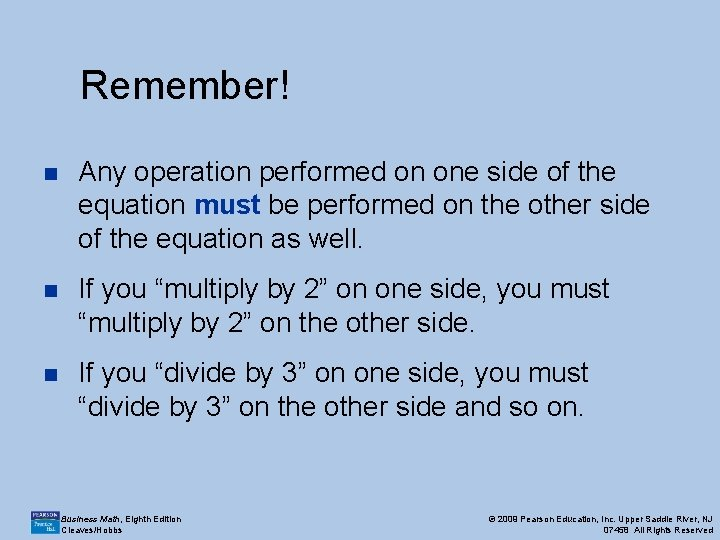Remember! n Any operation performed on one side of the equation must be performed on the other side of the equation as well. n If you “multiply by 2” on one side, you must “multiply by 2” on the other side. n If you “divide by 3” on one side, you must “divide by 3” on the other side and so on. Business Math, Eighth Edition Cleaves/Hobbs © 2009 Pearson Education, Inc. Upper Saddle River, NJ 07458 All Rights Reserved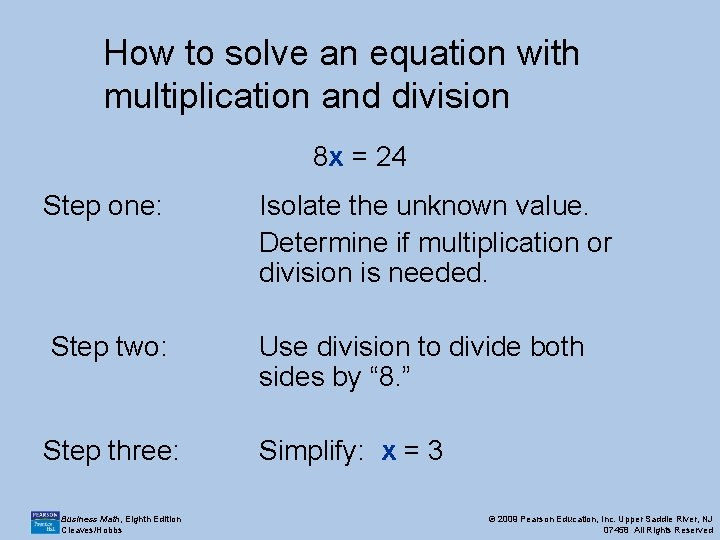How to solve an equation with multiplication and division 8 x = 24 Step one: Isolate the unknown value. Determine if multiplication or division is needed. Step two: Use division to divide both sides by “ 8. ” Step three: Simplify: x = 3 Business Math, Eighth Edition Cleaves/Hobbs © 2009 Pearson Education, Inc. Upper Saddle River, NJ 07458 All Rights Reserved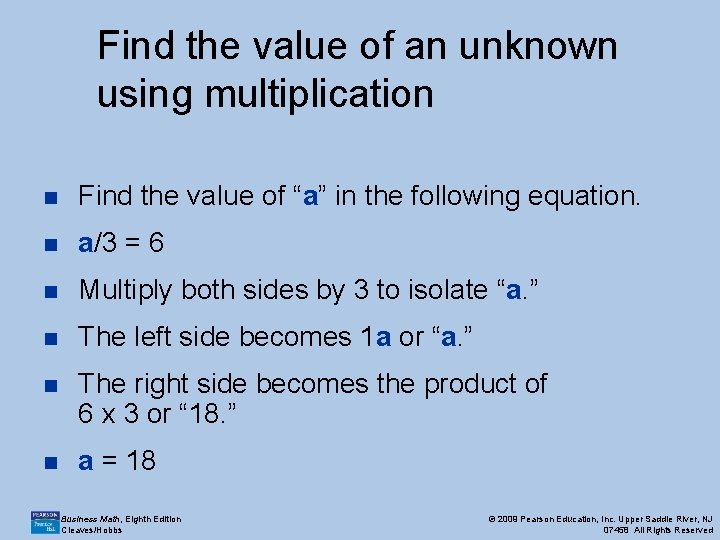Find the value of an unknown using multiplication n Find the value of “a” in the following equation. n a/3 = 6 n Multiply both sides by 3 to isolate “a. ” n The left side becomes 1 a or “a. ” n The right side becomes the product of 6 x 3 or “ 18. ” n a = 18 Business Math, Eighth Edition Cleaves/Hobbs © 2009 Pearson Education, Inc. Upper Saddle River, NJ 07458 All Rights Reserved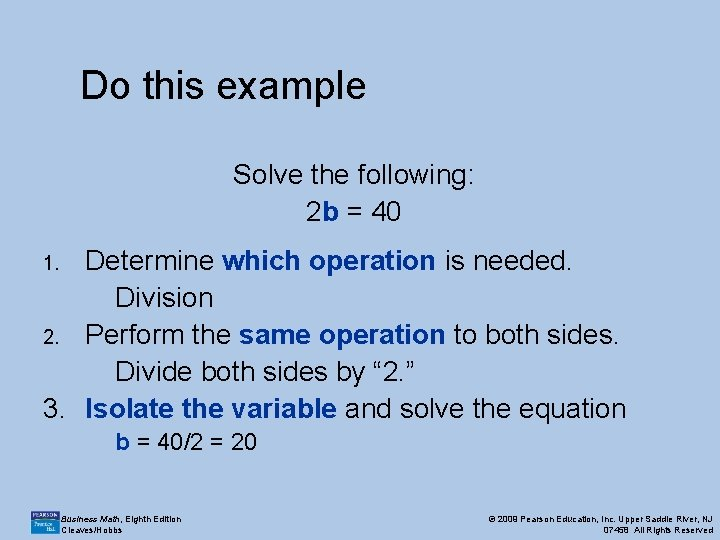Do this example Solve the following: 2 b = 40 Determine which operation is needed. Division 2. Perform the same operation to both sides. Divide both sides by “ 2. ” 3. Isolate the variable and solve the equation 1. b = 40/2 = 20 Business Math, Eighth Edition Cleaves/Hobbs © 2009 Pearson Education, Inc. Upper Saddle River, NJ 07458 All Rights Reserved5. 1. 2 Solve an Equation with Addition or Subtraction Step one: 4 + x = 10 Isolate the unknown value. Determine if addition or subtraction is needed. Step two: Use subtraction to isolate “x. ” Step three: Simplify: x = 6 Business Math, Eighth Edition Cleaves/Hobbs © 2009 Pearson Education, Inc. Upper Saddle River, NJ 07458 All Rights Reserved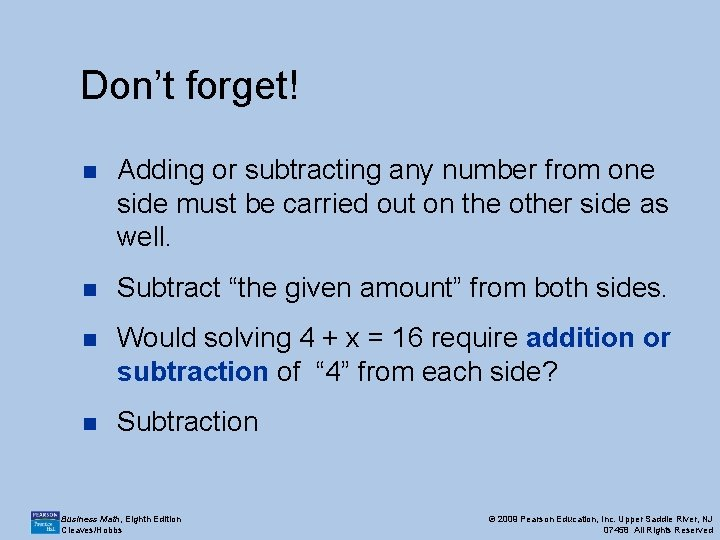Don’t forget! n Adding or subtracting any number from one side must be carried out on the other side as well. n Subtract “the given amount” from both sides. n Would solving 4 + x = 16 require addition or subtraction of “ 4” from each side? n Subtraction Business Math, Eighth Edition Cleaves/Hobbs © 2009 Pearson Education, Inc. Upper Saddle River, NJ 07458 All Rights Reserved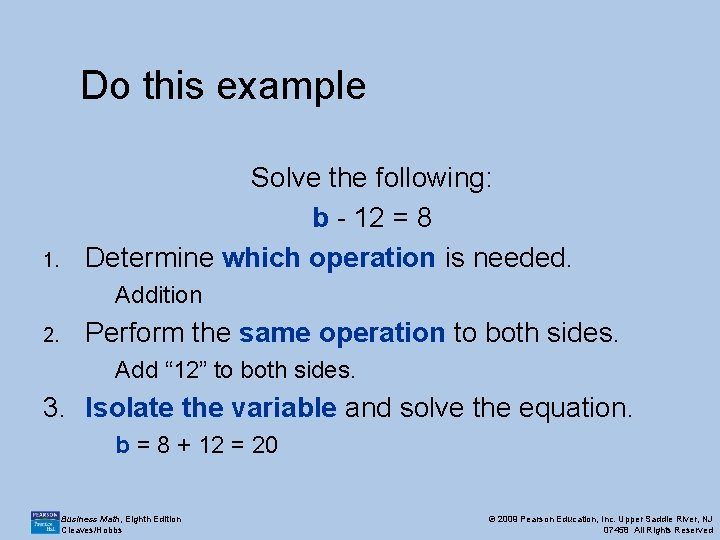Do this example 1. Solve the following: b - 12 = 8 Determine which operation is needed. Addition 2. Perform the same operation to both sides. Add “ 12” to both sides. 3. Isolate the variable and solve the equation. b = 8 + 12 = 20 Business Math, Eighth Edition Cleaves/Hobbs © 2009 Pearson Education, Inc. Upper Saddle River, NJ 07458 All Rights Reserved5. 1. 3 Solve Equations Using More Than One Operation n Isolate the unknown value. ¡ Add or subtract as necessary first. ¡ Multiply or divide as necessary second. n Identify the solution: the number on the side opposite the unknown. n Check the solution by “plugging in” the number using the original equation. Business Math, Eighth Edition Cleaves/Hobbs © 2009 Pearson Education, Inc. Upper Saddle River, NJ 07458 All Rights ReservedOrder of Operations n When two or more calculations are written symbolically, it is agreed to perform the operations according to a specified order of operations. n Perform multiplication and division as they appear from left to right. n Perform addition and subtraction as they appear from left to right. Business Math, Eighth Edition Cleaves/Hobbs © 2009 Pearson Education, Inc. Upper Saddle River, NJ 07458 All Rights Reserved“Undo the operations” To solve an equation, we undo the operations, so we work in reverse order. 1. Undo the addition or subtraction. 2. Undo multiplication or division. 7 x + 4 = 39 Business Math, Eighth Edition Cleaves/Hobbs © 2009 Pearson Education, Inc. Upper Saddle River, NJ 07458 All Rights Reserved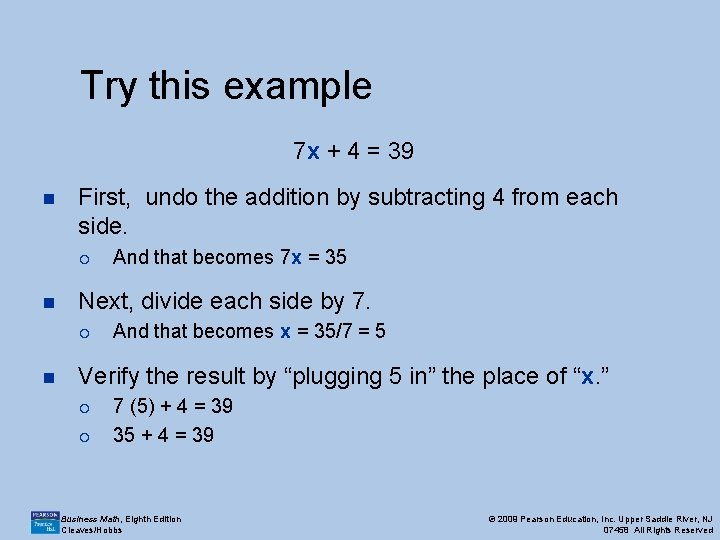Try this example 7 x + 4 = 39 n First, undo the addition by subtracting 4 from each side. ¡ n Next, divide each side by 7. ¡ n And that becomes 7 x = 35 And that becomes x = 35/7 = 5 Verify the result by “plugging 5 in” the place of “x. ” ¡ ¡ 7 (5) + 4 = 39 35 + 4 = 39 Business Math, Eighth Edition Cleaves/Hobbs © 2009 Pearson Education, Inc. Upper Saddle River, NJ 07458 All Rights Reserved5. 1. 4 Equations Containing Multiple Unknown Terms n In some equations, the unknown value may occur more than once. n The simplest instance is when the unknown value occurs in two addends. n For example: 3 a + 2 a = 25 n Add the numbers in each addend (2+3). n Multiply the sum by the unknown (5 a = 25). n Solve for “a. ” (a = 5) Business Math, Eighth Edition Cleaves/Hobbs © 2009 Pearson Education, Inc. Upper Saddle River, NJ 07458 All Rights Reserved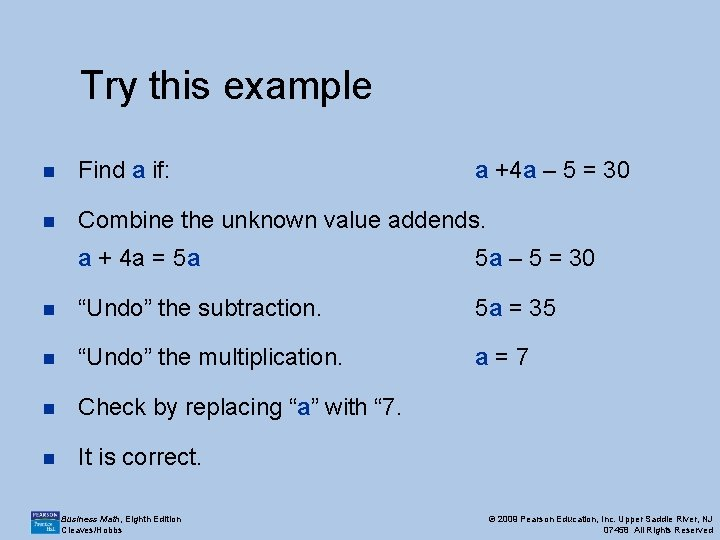Try this example n Find a if: a +4 a – 5 = 30 n Combine the unknown value addends. a + 4 a = 5 a 5 a – 5 = 30 n “Undo” the subtraction. 5 a = 35 n “Undo” the multiplication. a=7 n Check by replacing “a” with “ 7. n It is correct. Business Math, Eighth Edition Cleaves/Hobbs © 2009 Pearson Education, Inc. Upper Saddle River, NJ 07458 All Rights Reserved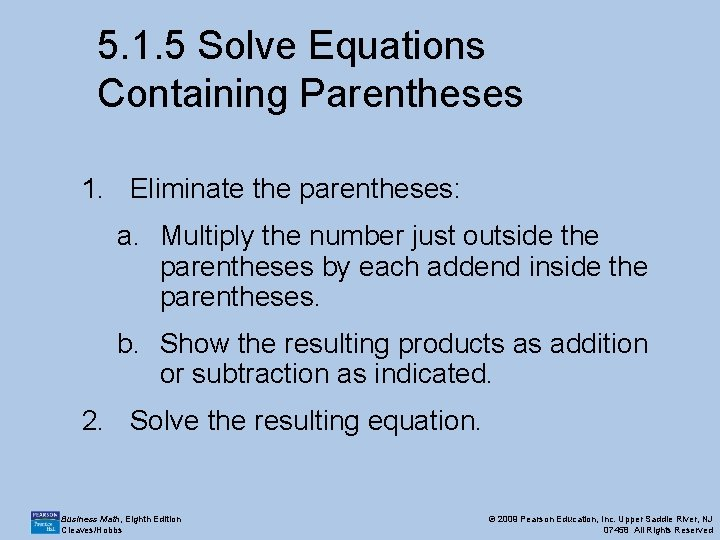5. 1. 5 Solve Equations Containing Parentheses 1. Eliminate the parentheses: a. Multiply the number just outside the parentheses by each addend inside the parentheses. b. Show the resulting products as addition or subtraction as indicated. 2. Solve the resulting equation. Business Math, Eighth Edition Cleaves/Hobbs © 2009 Pearson Education, Inc. Upper Saddle River, NJ 07458 All Rights Reserved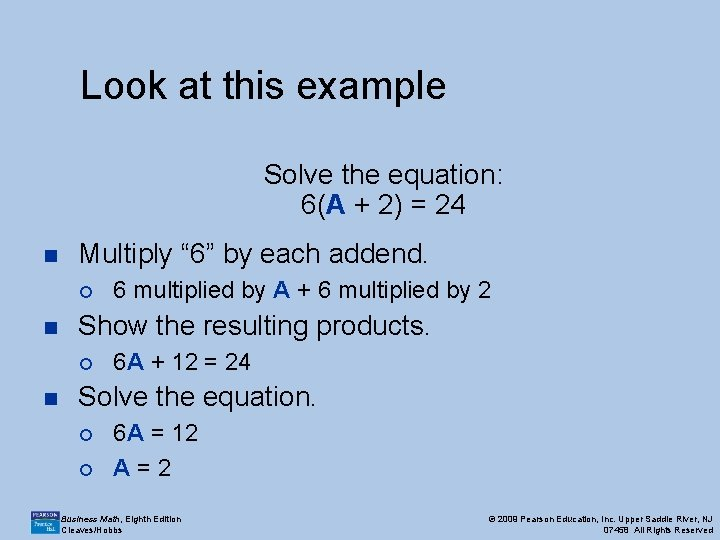Look at this example Solve the equation: 6(A + 2) = 24 n Multiply “ 6” by each addend. ¡ n Show the resulting products. ¡ n 6 multiplied by A + 6 multiplied by 2 6 A + 12 = 24 Solve the equation. ¡ ¡ 6 A = 12 A=2 Business Math, Eighth Edition Cleaves/Hobbs © 2009 Pearson Education, Inc. Upper Saddle River, NJ 07458 All Rights Reserved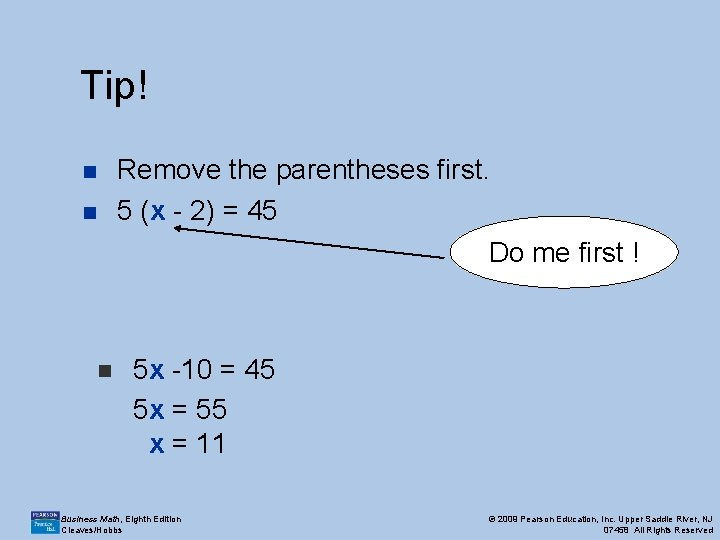Tip! Remove the parentheses first. 5 (x - 2) = 45 n n Do me first ! n 5 x -10 = 45 5 x = 55 x = 11 Business Math, Eighth Edition Cleaves/Hobbs © 2009 Pearson Education, Inc. Upper Saddle River, NJ 07458 All Rights Reserved5. 1. 6 Solve Equations That are Proportions n A proportion is based on two pairs of related quantities. n The most common way to write proportions is to use fraction notation. n A number written in fraction notation is also called a ratio. n When two ratios are equal, they form a proportion. Business Math, Eighth Edition Cleaves/Hobbs © 2009 Pearson Education, Inc. Upper Saddle River, NJ 07458 All Rights Reserved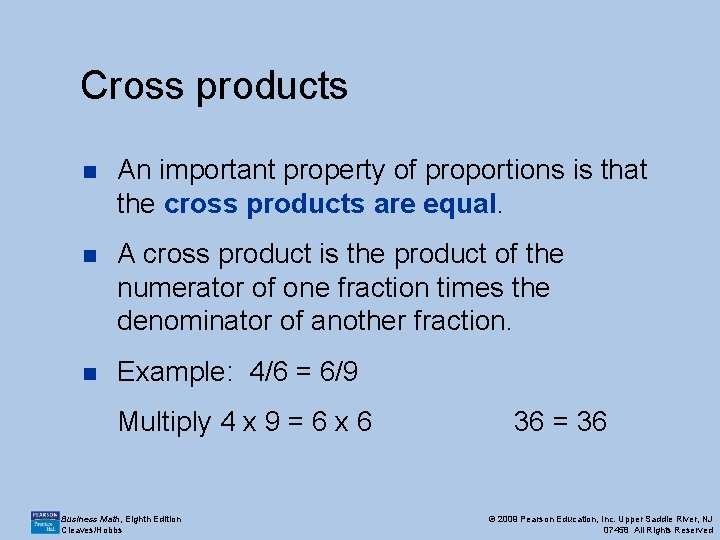Cross products n An important property of proportions is that the cross products are equal. n A cross product is the product of the numerator of one fraction times the denominator of another fraction. n Example: 4/6 = 6/9 Multiply 4 x 9 = 6 x 6 Business Math, Eighth Edition Cleaves/Hobbs 36 = 36 © 2009 Pearson Education, Inc. Upper Saddle River, NJ 07458 All Rights Reserved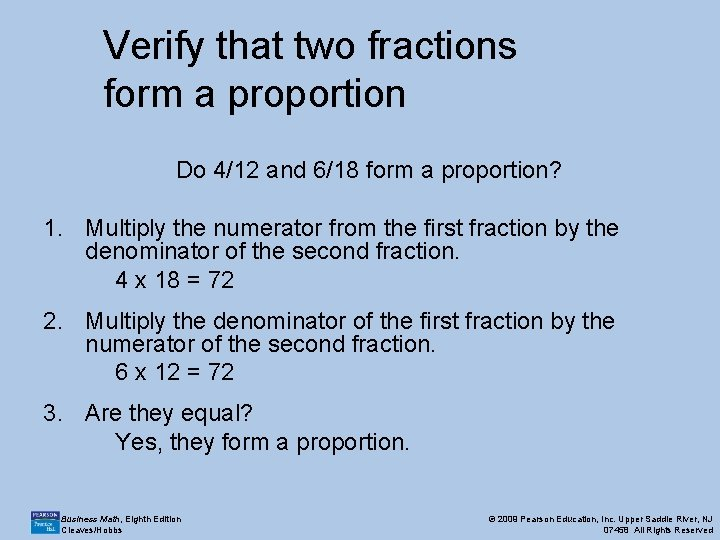Verify that two fractions form a proportion Do 4/12 and 6/18 form a proportion? 1. Multiply the numerator from the first fraction by the denominator of the second fraction. 4 x 18 = 72 2. Multiply the denominator of the first fraction by the numerator of the second fraction. 6 x 12 = 72 3. Are they equal? Yes, they form a proportion. Business Math, Eighth Edition Cleaves/Hobbs © 2009 Pearson Education, Inc. Upper Saddle River, NJ 07458 All Rights Reserved5. 2 Using Equations to Solve Problems n There is a list of key words and what operations they imply in your textbook. Please refer to it. n These words help you interpret the information and begin to set up the equation to solve the problem. n Example: “of” often implies multiplication. “¼ of her salary” means “multiply her salary by ¼” Business Math, Eighth Edition Cleaves/Hobbs © 2009 Pearson Education, Inc. Upper Saddle River, NJ 07458 All Rights ReservedFive-step problem solving approach for equations n What you know. ¡ n What you are looking for? ¡ n Equation or relationship among known / unknown facts. Solution ¡ n Unknown or missing amounts. Solution Plan ¡ n Known or given facts. Solve the equation. Conclusion ¡ Solution interpreted within context of problem. Business Math, Eighth Edition Cleaves/Hobbs © 2009 Pearson Education, Inc. Upper Saddle River, NJ 07458 All Rights Reserved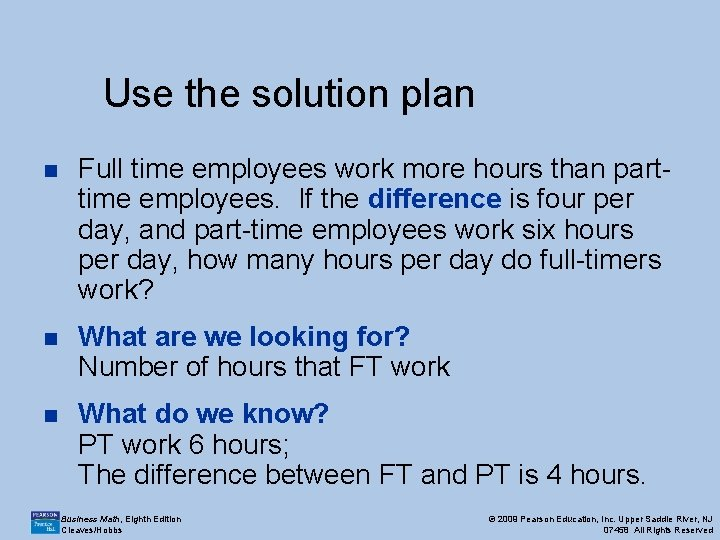Use the solution plan n Full time employees work more hours than parttime employees. If the difference is four per day, and part-time employees work six hours per day, how many hours per day do full-timers work? n What are we looking for? Number of hours that FT work n What do we know? PT work 6 hours; The difference between FT and PT is 4 hours. Business Math, Eighth Edition Cleaves/Hobbs © 2009 Pearson Education, Inc. Upper Saddle River, NJ 07458 All Rights Reserved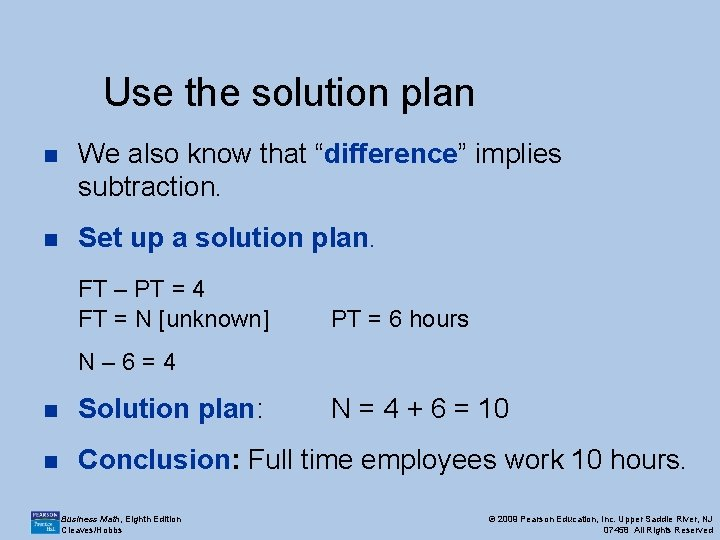Use the solution plan n We also know that “difference” implies subtraction. n Set up a solution plan. FT – PT = 4 FT = N [unknown] PT = 6 hours N– 6=4 n Solution plan: n Conclusion: Full time employees work 10 hours. Business Math, Eighth Edition Cleaves/Hobbs N = 4 + 6 = 10 © 2009 Pearson Education, Inc. Upper Saddle River, NJ 07458 All Rights Reserved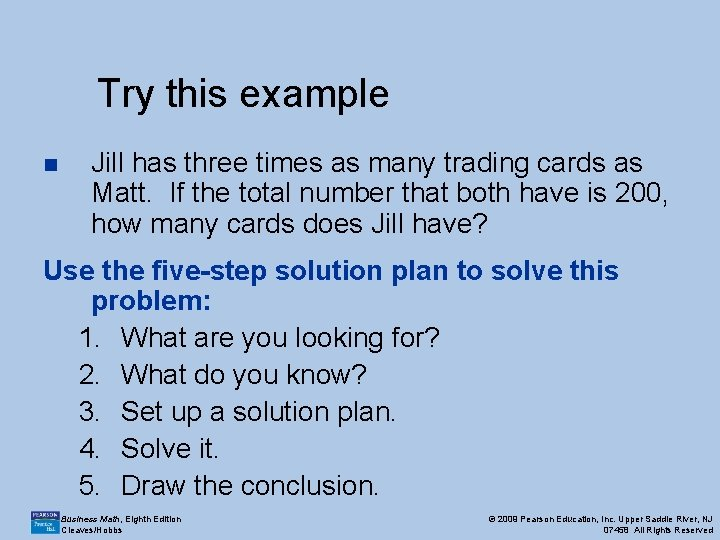Try this example n Jill has three times as many trading cards as Matt. If the total number that both have is 200, how many cards does Jill have? Use the five-step solution plan to solve this problem: 1. What are you looking for? 2. What do you know? 3. Set up a solution plan. 4. Solve it. 5. Draw the conclusion. Business Math, Eighth Edition Cleaves/Hobbs © 2009 Pearson Education, Inc. Upper Saddle River, NJ 07458 All Rights Reserved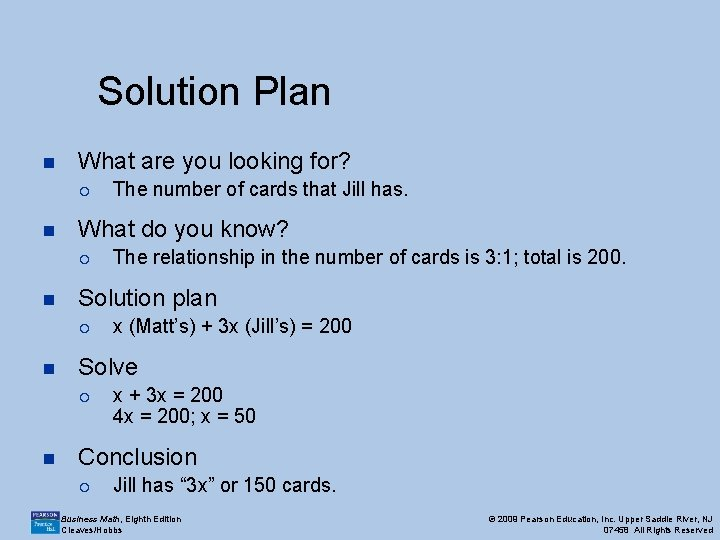Solution Plan n What are you looking for? ¡ n What do you know? ¡ n x (Matt’s) + 3 x (Jill’s) = 200 Solve ¡ n The relationship in the number of cards is 3: 1; total is 200. Solution plan ¡ n The number of cards that Jill has. x + 3 x = 200 4 x = 200; x = 50 Conclusion ¡ Jill has “ 3 x” or 150 cards. Business Math, Eighth Edition Cleaves/Hobbs © 2009 Pearson Education, Inc. Upper Saddle River, NJ 07458 All Rights ReservedSolving a word problem with a total of two types of items Diane’s Card Shop spent a total of \$950 ordering 600 cards from Wit’s End Co. , whose humorous cards cost \$1. 75 each and whose nature cards cost \$1. 50 each. How many of each style of card did the card shop order? n Use the solution plan to solve this problem. Business Math, Eighth Edition Cleaves/Hobbs © 2009 Pearson Education, Inc. Upper Saddle River, NJ 07458 All Rights Reserved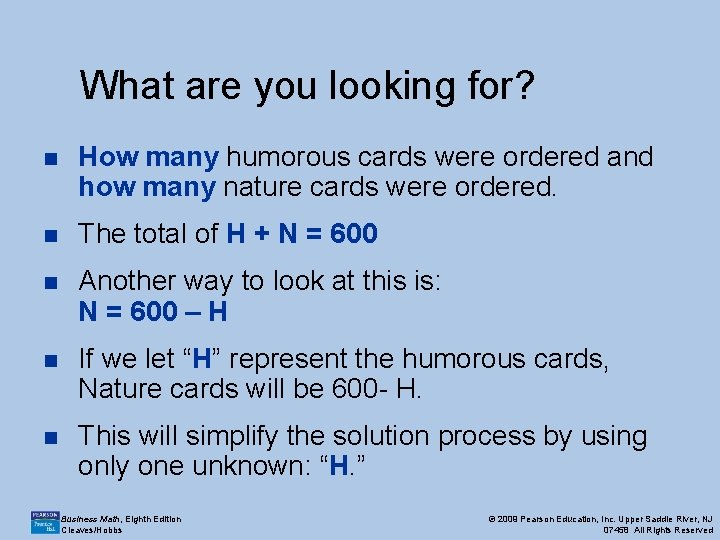What are you looking for? n How many humorous cards were ordered and how many nature cards were ordered. n The total of H + N = 600 n Another way to look at this is: N = 600 – H n If we let “H” represent the humorous cards, Nature cards will be 600 - H. n This will simplify the solution process by using only one unknown: “H. ” Business Math, Eighth Edition Cleaves/Hobbs © 2009 Pearson Education, Inc. Upper Saddle River, NJ 07458 All Rights Reserved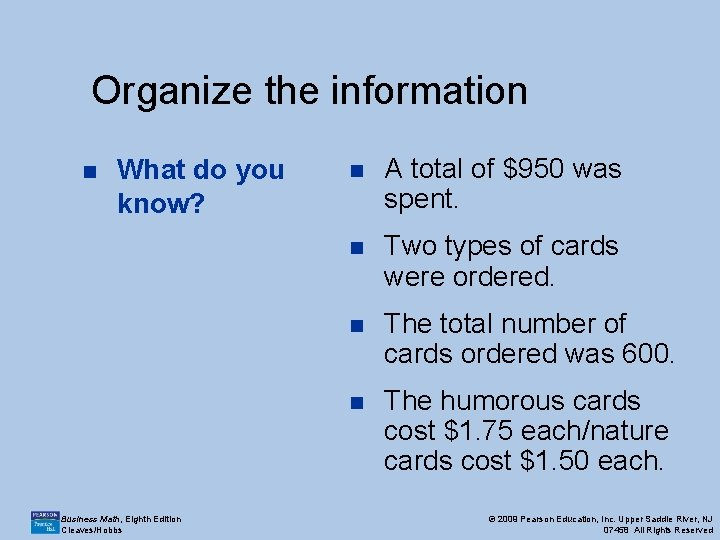Organize the information n What do you know? Business Math, Eighth Edition Cleaves/Hobbs n A total of \$950 was spent. n Two types of cards were ordered. n The total number of cards ordered was 600. n The humorous cards cost \$1. 75 each/nature cards cost \$1. 50 each. © 2009 Pearson Education, Inc. Upper Saddle River, NJ 07458 All Rights Reserved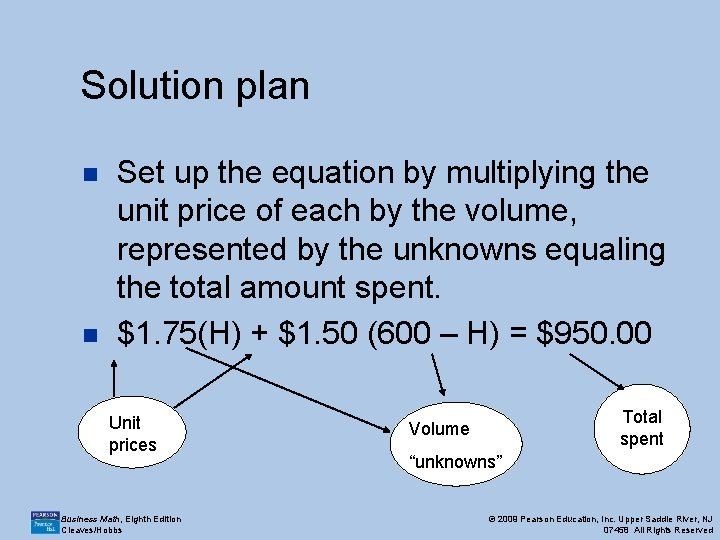Solution plan n n Set up the equation by multiplying the unit price of each by the volume, represented by the unknowns equaling the total amount spent. \$1. 75(H) + \$1. 50 (600 – H) = \$950. 00 Unit prices Business Math, Eighth Edition Cleaves/Hobbs Total spent Volume “unknowns” © 2009 Pearson Education, Inc. Upper Saddle River, NJ 07458 All Rights ReservedSolve the equation n \$1. 75 H + \$1. 50(600 -H) = \$950. 00 n \$1. 75 H + \$900. 00 - \$1. 50 H = \$950. 00 n \$0. 25 H + \$900. 00 = \$950. 00 n \$0. 25 H = \$50. 00 n H = 200 Business Math, Eighth Edition Cleaves/Hobbs © 2009 Pearson Education, Inc. Upper Saddle River, NJ 07458 All Rights Reserved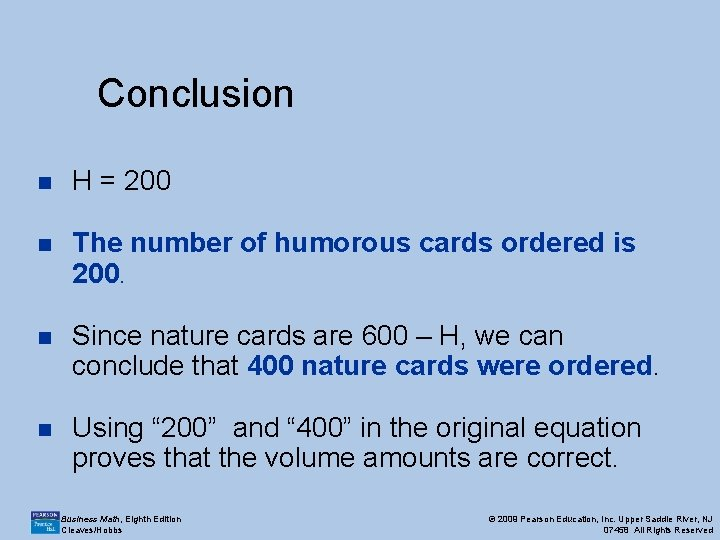Conclusion n H = 200 n The number of humorous cards ordered is 200. n Since nature cards are 600 – H, we can conclude that 400 nature cards were ordered. n Using “ 200” and “ 400” in the original equation proves that the volume amounts are correct. Business Math, Eighth Edition Cleaves/Hobbs © 2009 Pearson Education, Inc. Upper Saddle River, NJ 07458 All Rights Reserved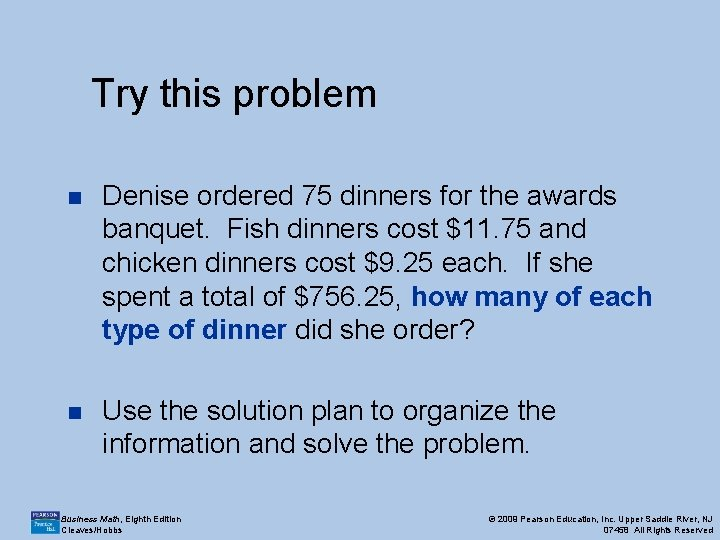Try this problem n Denise ordered 75 dinners for the awards banquet. Fish dinners cost \$11. 75 and chicken dinners cost \$9. 25 each. If she spent a total of \$756. 25, how many of each type of dinner did she order? n Use the solution plan to organize the information and solve the problem. Business Math, Eighth Edition Cleaves/Hobbs © 2009 Pearson Education, Inc. Upper Saddle River, NJ 07458 All Rights ReservedDenise’s order n \$11. 75(F) + \$9. 25(75 -F) = \$756. 25 n \$11. 75 F + \$693. 75 - \$9. 25 F = \$756. 25 n \$2. 50 F + \$693. 75 = \$756. 25 n \$2. 50 F = \$62. 50 n F = 25 n Conclusion: 25 fish dinners and 50 chicken dinners were ordered. Business Math, Eighth Edition Cleaves/Hobbs © 2009 Pearson Education, Inc. Upper Saddle River, NJ 07458 All Rights Reserved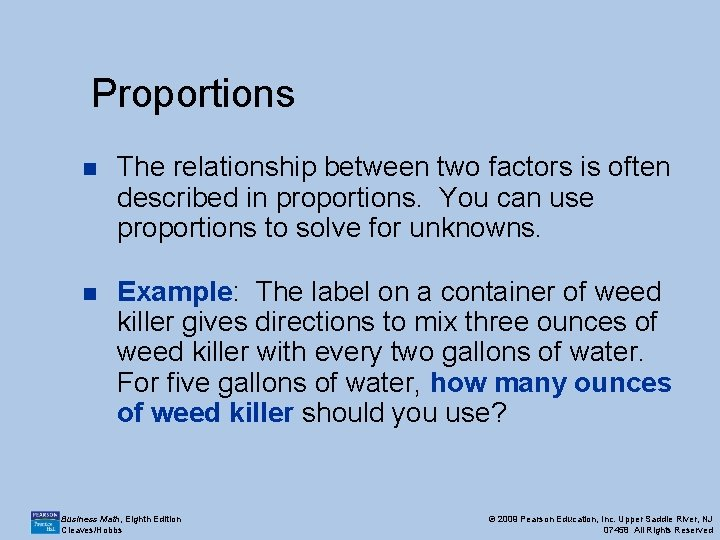Proportions n The relationship between two factors is often described in proportions. You can use proportions to solve for unknowns. n Example: The label on a container of weed killer gives directions to mix three ounces of weed killer with every two gallons of water. For five gallons of water, how many ounces of weed killer should you use? Business Math, Eighth Edition Cleaves/Hobbs © 2009 Pearson Education, Inc. Upper Saddle River, NJ 07458 All Rights Reserved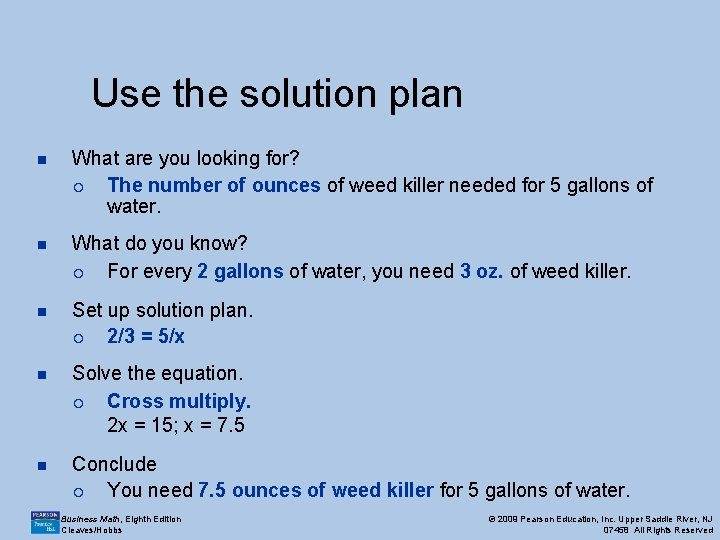Use the solution plan n What are you looking for? ¡ The number of ounces of weed killer needed for 5 gallons of water. n What do you know? ¡ For every 2 gallons of water, you need 3 oz. of weed killer. n Set up solution plan. ¡ 2/3 = 5/x n Solve the equation. ¡ Cross multiply. 2 x = 15; x = 7. 5 n Conclude ¡ You need 7. 5 ounces of weed killer for 5 gallons of water. Business Math, Eighth Edition Cleaves/Hobbs © 2009 Pearson Education, Inc. Upper Saddle River, NJ 07458 All Rights Reserved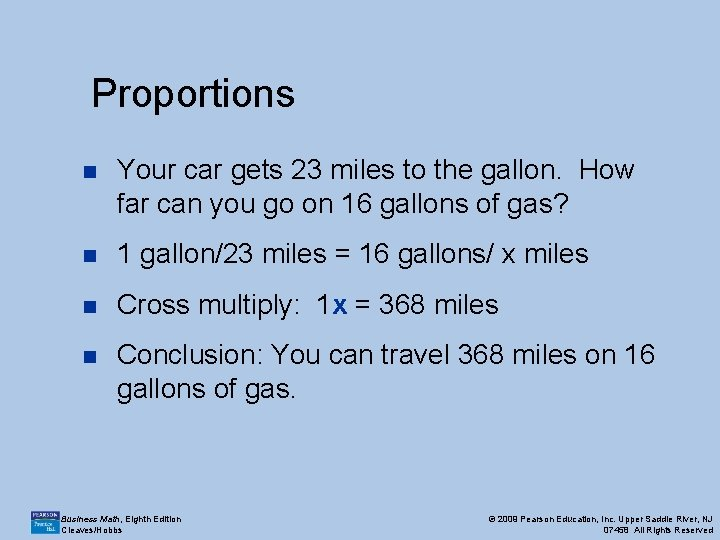Proportions n Your car gets 23 miles to the gallon. How far can you go on 16 gallons of gas? n 1 gallon/23 miles = 16 gallons/ x miles n Cross multiply: 1 x = 368 miles n Conclusion: You can travel 368 miles on 16 gallons of gas. Business Math, Eighth Edition Cleaves/Hobbs © 2009 Pearson Education, Inc. Upper Saddle River, NJ 07458 All Rights ReservedDirect Proportions n Many business-related problems that involve pairs of numbers that are proportional involve direct proportions. n An increase (or decrease) in one amount causes an increase (or decrease) in the number that pairs with it. n In the previous example, an increase in the amount of gas would directly and proportionately increase the mileage yielded. Business Math, Eighth Edition Cleaves/Hobbs © 2009 Pearson Education, Inc. Upper Saddle River, NJ 07458 All Rights Reserved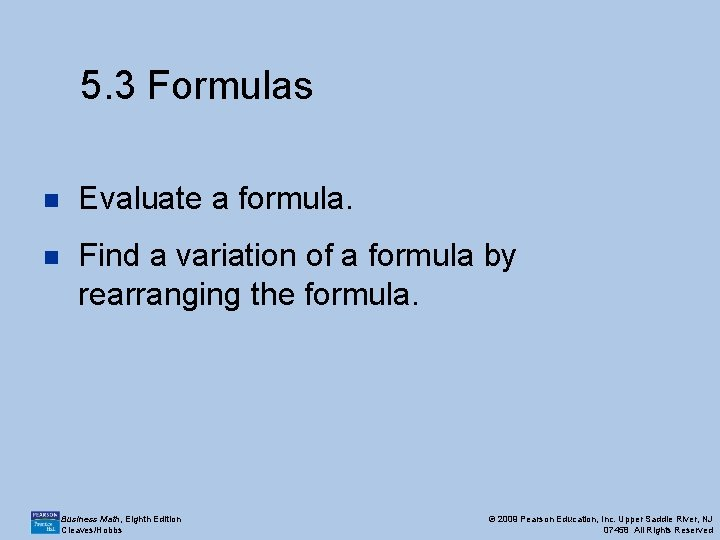5. 3 Formulas n Evaluate a formula. n Find a variation of a formula by rearranging the formula. Business Math, Eighth Edition Cleaves/Hobbs © 2009 Pearson Education, Inc. Upper Saddle River, NJ 07458 All Rights ReservedHow to evaluate a formula n Write the formula. n Rewrite the formula substituting known values for the letters of the formula. n Solve the equation for the unknown letter or perform the indicated operations, applying the order of operations. n Interpret the solution within the context of the formula. Business Math, Eighth Edition Cleaves/Hobbs © 2009 Pearson Education, Inc. Upper Saddle River, NJ 07458 All Rights Reserved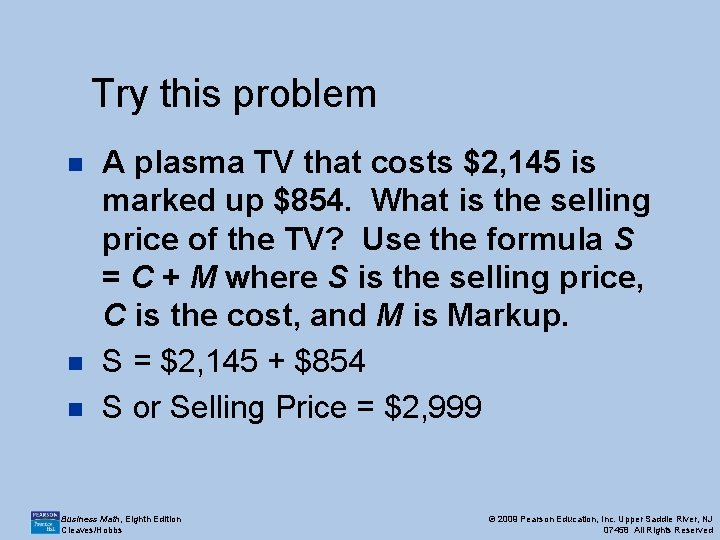Try this problem n n n A plasma TV that costs \$2, 145 is marked up \$854. What is the selling price of the TV? Use the formula S = C + M where S is the selling price, C is the cost, and M is Markup. S = \$2, 145 + \$854 S or Selling Price = \$2, 999 Business Math, Eighth Edition Cleaves/Hobbs © 2009 Pearson Education, Inc. Upper Saddle River, NJ 07458 All Rights ReservedFind a variation of a formula by rearranging the formula n Determine which variable of the formula is to be isolated (solved for). n Highlight or mentally locate all instances of the variable to be isolated. n Treat all other variables of the formula as you would treat numbers in an equation, and perform normal steps for solving an equation. n If the isolated variable is on the right side of the equation, interchange the sides so that it appears on the left side. Business Math, Eighth Edition Cleaves/Hobbs © 2009 Pearson Education, Inc. Upper Saddle River, NJ 07458 All Rights Reserved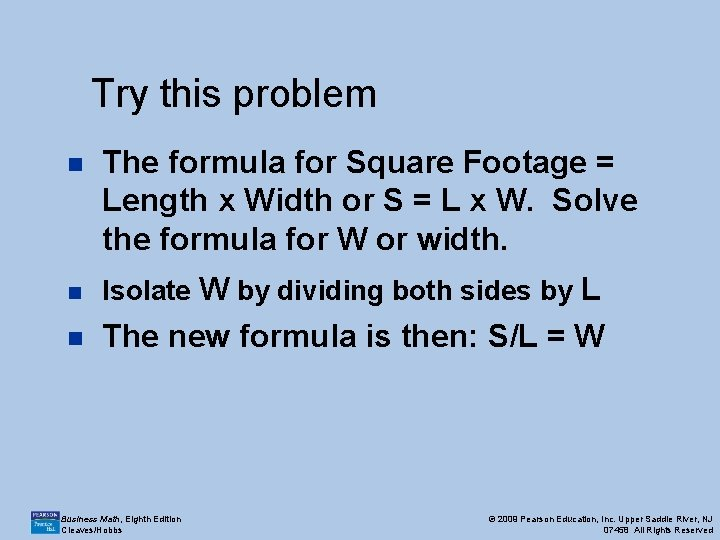Try this problem n The formula for Square Footage = Length x Width or S = L x W. Solve the formula for W or width. n Isolate W by dividing both sides by L n The new formula is then: S/L = W Business Math, Eighth Edition Cleaves/Hobbs © 2009 Pearson Education, Inc. Upper Saddle River, NJ 07458 All Rights Reserved